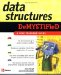# Quiz

 1 What is an abstract data type?2 What abstract data type would be used to store a whole number?3 Explain how a memory address is used to access an abstract data type that is larger than 1 byte.4 What is the difference between a float abstract data type and a double abstract data type?5 What is precision?6 Explain how memory is organized within a computer.7 What is a numbering system?8 Why is the binary numbering system used in computing?9 Why don t you directly specify the number of bytes to reserve in memory to store data?10 Explain the impact signed and unsigned numbers have on memory.1 An abstract data type is a keyword of a programming language that specifies the amount of memory needed to store data and the kind of data that will be stored in that memory location. 2 An integer: byte , short , int , or long . 3 Each memory address represents 1 byte of memory. Some abstract data types, such as an int , reserve 2 bytes of memory. Technically, data stored in this memory location has two memory address: one address for the first byte of memory and another address for the second byte of memory. However, the computer references only the address of the first byte of memory when accessing that memory location. 4 The double abstract data type is used to store real numbers that are very large or very small and require double the amount of memory that is reserved with a float abstract data type. 5 Precision refers the accuracy of the decimal portion of a value. 6 Memory consists of a series of switches called transistors . Each transistor stores a binary digit (bit). Transistors are logically organized into groups of 8 switches called a byte. Each byte is uniquely identified by a memory address. 7 A numbering system is a logical method used to count and perform arithmetic using digits to represent items. Each numbering system has a different number of digits. The decimal numbering systems has 10 digits, from 0 through 9. The binary numbering systems has 2 digits, 0 and 1. All numbering systems can be used to count and perform arithmetic, regardless of the number of digits contained in the numbering system. 8 The binary numbering system is used in computing because it contains 2 digits that can be stored by changing the state of a transistor. Off represents 0 and On represents 1. 9 A programmer doesn t specify the exact number of bytes to reserve in memory because the computer language determines the most efficient number of bytes to represent a data type. 10 The sign takes up 1 bit of memory that could otherwise be used to represent a value. For example, a byte has 8 bits, all of which can be used to store an unsigned number from 0 to 255. You can store a signed number in the range of “128 to +127.Data Structures Demystified (Demystified)
ISBN: 0072253592
EAN: 2147483647
Year: 2006
Pages: 90

Similar book on Amazon